Courses

# Numerical Control Of Machine Tools - 2

## 10 Questions MCQ Test Manufacturing and Industrial Engineering | Numerical Control Of Machine Tools - 2

Description
This mock test of Numerical Control Of Machine Tools - 2 for Mechanical Engineering helps you for every Mechanical Engineering entrance exam. This contains 10 Multiple Choice Questions for Mechanical Engineering Numerical Control Of Machine Tools - 2 (mcq) to study with solutions a complete question bank. The solved questions answers in this Numerical Control Of Machine Tools - 2 quiz give you a good mix of easy questions and tough questions. Mechanical Engineering students definitely take this Numerical Control Of Machine Tools - 2 exercise for a better result in the exam. You can find other Numerical Control Of Machine Tools - 2 extra questions, long questions & short questions for Mechanical Engineering on EduRev as well by searching above.
QUESTION: 1

### Match the List-I (Preparatory function codes) with List-II (Related functions):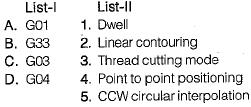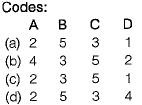Solution: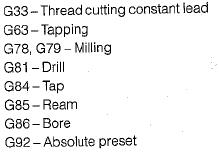QUESTION: 2

Solution:
QUESTION: 3

### Machining center is

Solution:
QUESTION: 4

In APT language, the cutter motion in incremental coordinate mode is addressed as

Solution:
QUESTION: 5

Suppose point P1 in APT (Automatically programmed tool) programming is coded by statement:
P= POINT/XSMALL, INTOF, LN1, CR1
The coded geometric situation without causing error is

Solution:
QUESTION: 6

The interpolator in a CNC machine controls

Solution:
QUESTION: 7

In a CNC machine feed drive, a stepper motor with step angle of 1.80 drives a lead screw with pitch of 2 mm. The basic length unit (BLU) for this drive is

Solution:
QUESTION: 8

In a CAD package, mirror image of a 2D point P(5, 10) is to be obtained about a line which passes through the origin and makes an angle of 450 counterclockwise with the X-axis. The coordinates of the transformed point will be

Solution: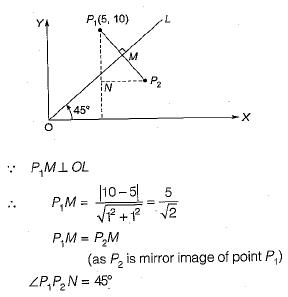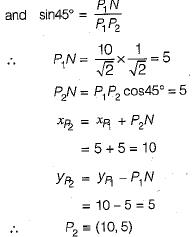QUESTION: 9

Feed drives in CNC milling are provided by

Solution:
QUESTION: 10

Transfer machines can be defined as

Solution: